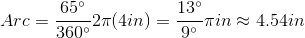# ISEE Upper Level Math : How to find the length of an arc

## Example Questions

### Example Question #1 : How To Find The Length Of An Arc

A giant clock has a minute hand six feet long. How far, in inches, did the tip move between noon and 1:20 PM?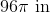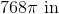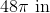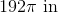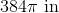Explanation:

The distance that the tip of the minute hand moves during one hour is the circumference of a circle with radius 6 feet. This circumference is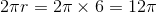feet. One hour and twenty minutes is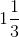hours, so the tip of the hand moved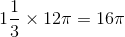feet, or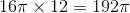inches.

### Example Question #2 : How To Find The Length Of An Arc

A giant clock has a minute hand three feet long. How far, in inches, did the tip move between noon and 12:20 PM?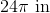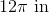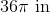It is impossible to tell from the information given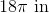Explanation:

The distance that the tip of the minute hand moves during one hour is the circumference of a circle with radiusfeet. This circumference is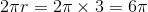feet.minutes is one-third of an hour, so the tip of the minute hand moves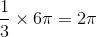feet, or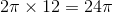inches.

### Example Question #3 : How To Find The Length Of An Arc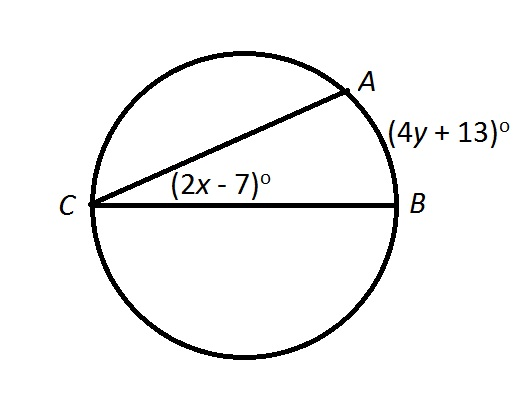In the above figure, expressin terms of.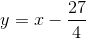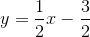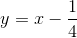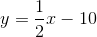Explanation:

The measure of an arc -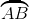- intercepted by an inscribed angle -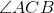- is twice the measure of that angle, so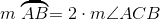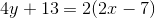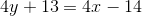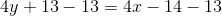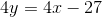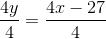### Example Question #4 : How To Find The Length Of An Arc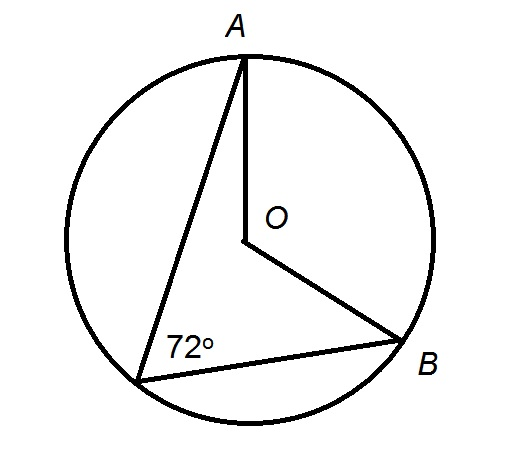In the above diagram, radius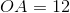.

Give the length of.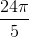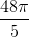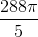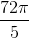Explanation:

The circumference of a circle ismultiplied by its radius , so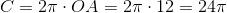., being an inscribed angle of the circle, intercepts an arcwith twice its measure: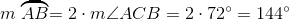The length ofis the circumference multiplied by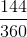: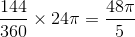.

### Example Question #5 : How To Find The Length Of An Arc

While visiting a history museum, you see a radar display which consists of a circular screen with a highlighted wedge with an angle of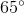. If the screen has a radius of 4 inches, what is the length of the arc of the highlighted wedge?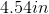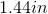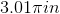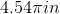Explanation:

While visiting a history museum, you see a radar display which consists of a circular screen with a highlighted wedge with an angle of. If the screen has a radius of 4 inches, what is the length of the arc of the highlighted wedge?

To begin, let's recall our formula for length of an arc.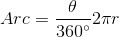Now, just plug in and simplify